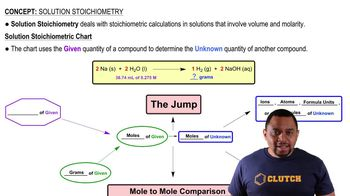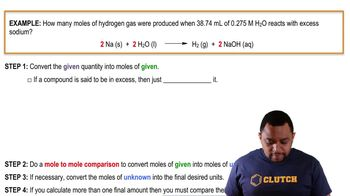Start typing, then use the up and down arrows to select an option from the list.
13:01 minutes
Problem 153a
Textbook Question

# Four solutions are prepared and mixed in the following order: (a) Start with 100.0 mL of 0.100 M BaCl2 (b) Add 50.0 mL of 0.100 M AgNO3 (c) Add 50.0 mL of 0.100 M H2SO4 (d) Add 250.0 mL of 0.100 M NH3. Write an equation for any reaction that occurs after each step, and calculate the concentrations of Ba2+, Cl-, NO3-, NH3, and NH4+ in the final solution, assuming that all reactions go to completion.Verified Solution
This video solution was recommended by our tutors as helpful for the problem above.
53views